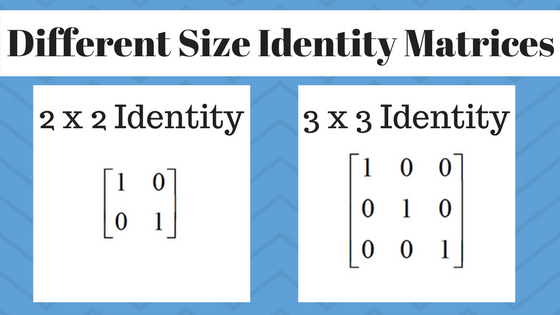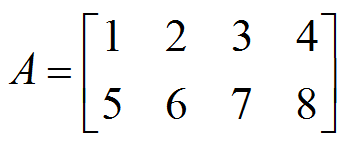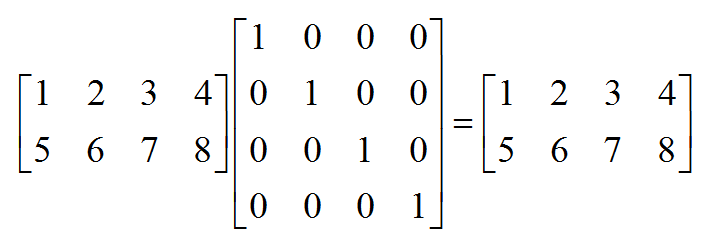﻿ The identity matrix and its properties - MathBootCamps

# The identity matrix and its properties

The identity matrix is a square matrix that has 1’s along the main diagonal and 0’s for all other entries. This matrix is often written simply as $$I$$, and is special in that it acts like 1 in matrix multiplication. In this lesson, we will look at this property and some other important idea associated with identity matrices.

## The identity matrix is always a square matrix

While we say “the identity matrix”, we are often talking about “an” identity matrix. For any whole number $$n$$, there is a corresponding $$n \times n$$ identity matrix. These matrices are said to be square since there is always the same number of rows and columns.To prevent confusion, a subscript is often used. So in the figure above, the $$2 \times 2$$ identity could be referred to as $$I_2$$ and the $$3 \times 3$$ identity could be referred to as $$I_3$$.

## Multiplying any matrix by the identity results in the matrix itself

When working with matrix multiplication, the size of a matrix is important as the multiplication is not always defined. Therefore for an $$m \times n$$ matrix $$A$$, we say:This shows that as long as the size of the matrix is considered, multiplying by the identity is like multiplying by 1 with numbers. For example, consider the following matrix.This is a $$2 \times 4$$ matrix since there are 2 rows and 4 columns. You can verify that $$I_2 A = A$$:and $$A I_4 = A$$:With other square matrices, this is much simpler. Consider the example below where $$B$$ is a $$2 \times 2$$ matrix. Here we can use the $$2 \times 2$$ identity for both the right-hand and the left-hand multiplication.## The product of two inverse matrices is always the identity

One concept studied heavily in mathematics is the concept of invertible matrices, which are those matrices that have an inverse. By definition, when you multiply two matrices that are inverses of each other, then you will get the identity matrix. Consider the following matrices:For these matrices, $$AB = BA = I$$, where $$I$$ is the $$2 \times 2$$ identity matrix.Therefore $$A$$ and $$B$$ are inverse matrices. You can study this idea more here: inverse matrices.

## Summary

The identity matrix is a fundamental idea when working with matrices – whether you are working with just multiplication, inverses, or even solving matrix equations. As you study these types of topics, be sure that you have a fundamental understanding of this matrix.## Subscribe to our Newsletter!

We are always posting new free lessons and adding more study guides, calculator guides, and problem packs.

Sign up to get occasional emails (once every couple or three weeks) letting you know what's new!